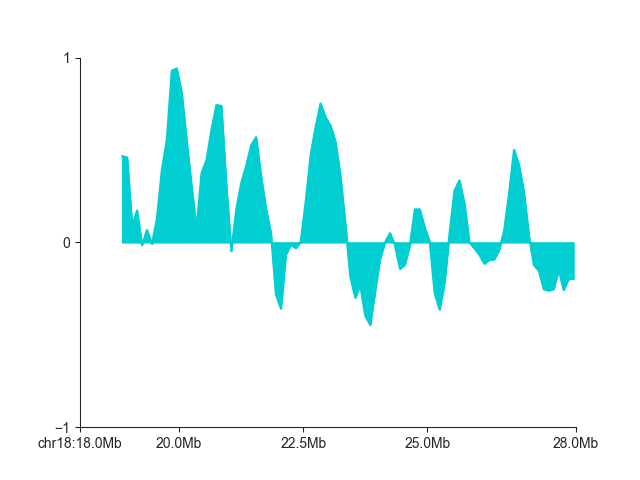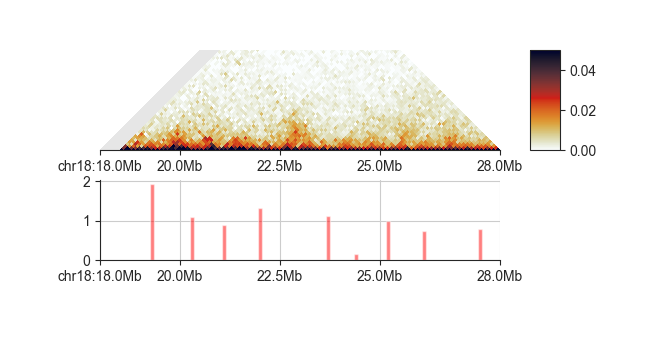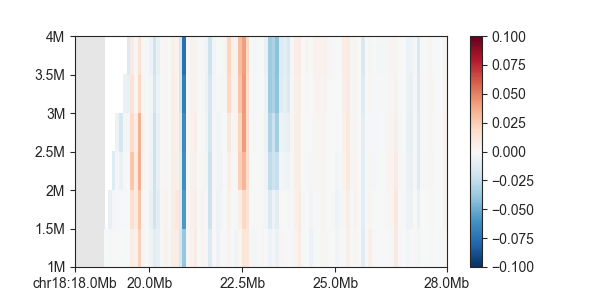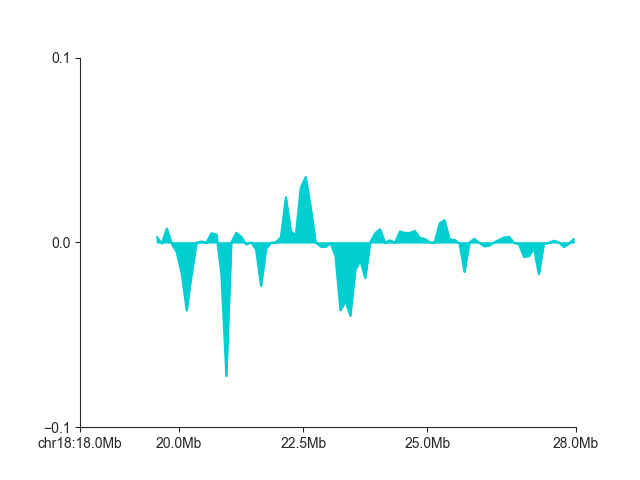To follow this tutorial, download the FAN-C example data, for example through our Keeper library. Then run the example `fanc auto` command in Example analysis to generate all the necessary files, and set up your Python session like this:

```import fanc
import fanc.plotting as fancplot
import logging

logging.basicConfig(level=logging.INFO, format="%(asctime)s %(levelname)s %(message)s")

```

If you want to try the tutorial with an equivalent Cooler file, load the Hi-C file like this instead:

```hic_100kb = fanc.load("architecture/other-hic/fanc_example.mcool@100kb")
```

or like this if you want to work with a Juicer file built from the same data:

```hic_100kb = fanc.load("architecture/other-hic/fanc_example.juicer.hic@100kb")
```

Note that there may be minor differences in the results due to the “zooming” and balancing applied by the different tools.

Also have a look at the command line documentation at Hi-C domain analysis, which explains a lot of the principles behind insulation scores and directionality indexes with a couple of helpful illustrations.

## Insulation scores¶

Insulation scores are calculated and saved to disk using the `InsulationScores` class. To run a basic insulation score calculation on a Hi-C object, use `from_hic`:

```insulation = fanc.InsulationScores.from_hic(hic_100kb,
[5000000, 1000000, 1500000,
2000000, 2500000, 3000000,
3500000, 4000000],
file_name="architecture/domains/fanc_example_100kb.insulation")
```

The file created in `file_name` can later be easily loaded with `load()`.

The resulting object stores all insulation score tracks for the `window_sizes` provided. It is derived from the `RegionsTable` class, which is `RegionBased`. There is a lot of convenient functionality bundled in these objects, which you can read about in RegionBased.

You can access the calculated scores by using the `scores()` function, which requires the window size of the scores you want to retrieve, and returns a list of scores, one for each genomic region in the object:

```scores = insulation.scores(1000000)
```

However, a more flexible way to retrieve scores, which allows things like subsetting and retrieving region information at the same time, is the `regions()` iterator. Scores are stored as a region attribute `insulation_<window size>`:

```for region in insulation.regions('chr18'):
score = region.insulation_1000000
# do something with score
# ...
```

If you prefer to have a dedicated object for a particular window size, you can use `score_regions()`. The score is then accessible via the `score` attribute:

```insulation_1mb = insulation.score_regions(1000000)
for r in insulation_1mb.regions:
score = r.score
```

### Insulation calculation parameters¶

`from_hic` has several parameters that allow you to customise the insulation score calculation. You can read everything them in the linked API reference, and we will highlight the most important ones here.

`impute_missing` allows you to replace missing or masked pixels with their expected values prior to the insulation score calculation. While this avoids dealing with missing pixels, this can be misleading in case larger regions are unmappable or have been filtered out for some other reason, so enable this setting with caution. The related parameter `na_threshold` instead controls how much of a sliding window can be covered by missing pixels before assigning it NaN by default. This is set to `0.5` by default - by increasing this value you will obtain more robust scores, but also the number of NaNs will increase in your results. Whatever value you choose, it is a tradeoff between information content and accuracy. In most cases you should be okay with the default setting.

`normalise`, which is enabled by default, divides insulation scores by their chromosome mean, so every score is expressed relative to the expected score on the same chromosome. By disabling this option, you will get raw sums of weights in the sliding window. Normalisation is stringly recommended, however. You can also normalise to a more local region instead of the whole chromosome, which may account for local variations in contact intensity other than insulation. For this purpose, set `normalisation_window` to a number of bins, for example `300`, which is then the number of scores surrounding each region used for normalisation by their mean.

After their computation and normalisation, insulation scores are log2-transformed. This makes scores (roughly) symmetrical around 0. To disable this, for example if you have also disabled `normalise`, use `log=False`.

The original insulation score definition uses the arithmetic mean to normalise the scores. If you expect insulation score outliers in your data, which might affect the normalisation by the mean, you can use a trimmed arithmetic mean instead by setting `trim_mean_proportion` to a value between 0 and 1. The top fraction of insulation scores is then discarded for calculating the mean.

If you intend to compare scores from different samples (and have the `normalise` and `log` options enabled), a mathematically more accurate normalisation would be to use the geometric mean instead. You can enable this by setting `geometric_mean=True`.

### Insulation score export¶

You can export scores to a human-readable file format using one of `to_bed()`, or `to_gff()`. Export to a fast BigWig track using `to_bigwig()`.

```insulation.to_bed("architecture/domains/fanc_example_100kb.insulation_1mb.bed", 1000000)
```

### Insulation score plots¶

You can plot all calculated insulation scores using `GenomicVectorArrayPlot`:

```p = fancplot.GenomicVectorArrayPlot(insulation, colormap='RdBu_r', vmin=-1, vmax=1,
genomic_format=True)
p.plot('chr18:18mb-28mb')
```If you only want to plot a single score track, use `LinePlot`:

```p = fancplot.LinePlot(insulation, ylim=(-1, 1), colors=['darkturquoise'], style="mid",
attribute="insulation_1000000")
p.plot('chr18:18mb-28mb')
```## Boundary calls¶

Once you have the `insulation` object, you can call insulating boundaries in the genome using `Boundaries`, specifically `from_insulation_score()`:

```boundaries = fanc.Boundaries.from_insulation_score(insulation, window_size=1000000)
```

This finds all minima in the insulation score vector and returns the corresponding regions as a `RegionsTable` object, which is RegionBased. The boundary strength is stored in the `score` attribute of each region:

```for boundary in boundaries.regions:
score = boundary.score
```

By default, all minima are reported, including very weak and likely false-positive boundaries. We recommend plotting the boundaries and their strength alongside the Hi-C matrix and then decide on a score cutoff to only select a robust set of boundaries!

```ph = fancplot.TriangularMatrixPlot(hic_100kb, max_dist=5000000, vmin=0, vmax=0.05)
pb = fancplot.BarPlot(boundaries)
f = fancplot.GenomicFigure([ph, pb])
fig, axes = f.plot('chr18:18mb-28mb')
```You can filter out false-positives manually like this

```min_score = 1.0
robust_boundaries = []
for boundary in boundaries.regions:
score = boundary.score
if score >= min_score:
robust_boundaries.append(boundary)
```

or by simply using the `min_score` argument:

```robust_boundaries = fanc.Boundaries.from_insulation_score(insulation, window_size=1000000,
min_score=1.0)
```

## Directionality index¶

For a short explanation of the directionality index, please read Directionality Index. The principle to compute the directionality index is highly similar to the insulation score. The dedicated class `DirectionalityIndexes` handles all relevant actions. Use `from_hic()` to compute it for multiple window sizes:

```directionality = fanc.DirectionalityIndexes.from_hic(hic_100kb,
[1000000, 1500000,
2000000, 2500000, 3000000,
3500000, 4000000],
file_name="architecture/domains/fanc_example_100kb.di")
```

Plotting also works the same way, for all indexes:

```p = fancplot.GenomicVectorArrayPlot(directionality, colormap='RdBu_r', vmin=-0.1, vmax=0.1,
genomic_format=True)
p.plot('chr18:18mb-28mb')
```And for single a single directionality index:

```p = fancplot.LinePlot(directionality, ylim=(-0.1, 0.1), colors=['darkturquoise'], style="mid",
attribute="directionality_4000000")
p.plot('chr18:18mb-28mb')
```